Start typing, then use the up and down arrows to select an option from the list.# Logarithmic Relationships

Jules Bruno
81views
1
1
Hey, guys. So here is gonna become important that you guys remember the different operations that occur, but we're dealing with log arrhythmic functions and natural algorithmic functions. So, for example, let's take a look. Multiplication. So here we have a log of a times B. Now that log is getting distributed to my A and my B because they're multiplying with each other. What that translates to is log of a plus log of B, and the same thing could be seen with our natural log. So, Ellen, a times B. Allen gets distributed to both because they're multiplying. It really means Allen of a plus, Allen of B. Next, we're dealing with division. So when we're dividing them, what does that really mean? Well, that means here that log of a divided by B becomes log of a minus log of the so when you're multiplying, it really means you're adding each one. But when you're dividing and subtracting same thing with my Ln Ln off a minus. Ellen of B. What happens when we raise it to a power? Well, when we raise it to a power, what you need to realize here is that this power can move up front of my log function. So this becomes X times long of a and same thing for Ln X comes up front. So becomes X equals a line of a. So there's a little bit of trick functions when it comes to these types of situations. This is gonna become important when we're dealing with chapters dealing with chemical kinetics. Because with those chapters, we do have to manipulate problems that contain Ln and then when it comes to P H and P o. H of acids and bases, that's when we have to take into account log functions. And some of the questions required these types of manipulations. Then if we're taking it to the 10th root. So here we have a log of X square root sign on a inside, remember That could be, too. That could be three. That could be four. So second route, which is square root, third root, fourth root. So remember what we're doing. A and Root, What does that really mean? First of all, what that means it's log off a to the one over x. Okay, so when you change that into a power becomes the reciprocal power so becomes one over X And again, Like we said before the expo, you can come up front. So this equals one over X Times log of a and then here's same thing with Ln it becomes Ln off a to the one over X again, this could come out front. So this equals one over x times Allen of a. So these are the different manipulations that you need to be aware off that will happen when we get to again chemical kinetics. And when we're dealing with log functions, off acids and bases based on what we've seen here, I say solve the phone without the use of a calculator. If log of three is equal to approximately 48 and log of two is equal to approximately 30 will be the value of log of 12. All right, so we have to think of which of these operations we can use. We want to find a log of 12 now an easy way, Thio to approach This is we could think of What could I do with three and to to give me a value off 12? That's why I gave you those numbers and the answer is if I do three times to that, gives me six, and then I find Multiply that by two again. That gives me 12 so we can say that Log of 12 can be seen as log off three times to times two, and we just saw up above. When you have things multiplying together, the law gets distributed to all of them. And because there's multiplication under being done, that really means it's log of three, plus log of to plus log of tip and soul. All we're gonna do now is they tell us what these values are. This is 48 this is 30 and this is 30 so we plug all that in. That gives me 1.8 as my final answer. So that would be my log of 12. And we did it without the use of a calculator, because we understand the different relationships that arise when we're dealing with log functions or natural log functions.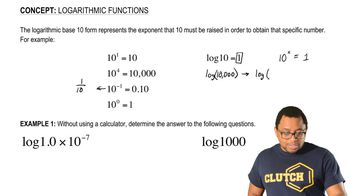03:37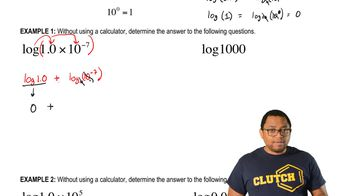01:34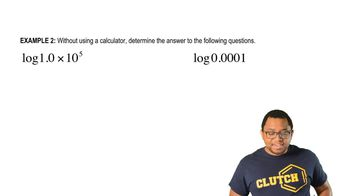02:16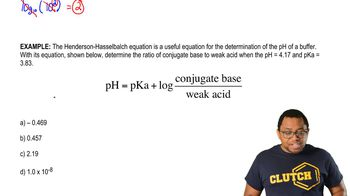04:24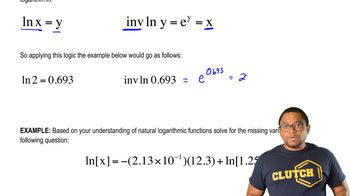03:54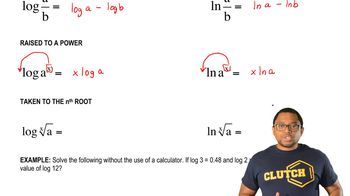04:56